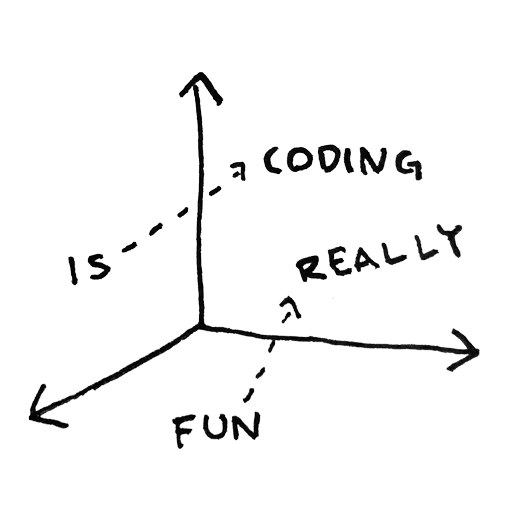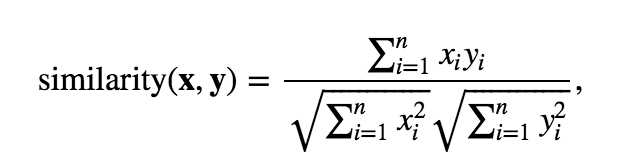Wait, but what on earth is a "Word Vector" 🤯? Learn more of these great tools for natural language processing e.g. here!# Use case example

So, what can I do with these features? You can for example assess similarity between words, or use them for classical machine learning classification problem. An example below to predict if a word is used in a News or Sports article.

## Cosine similarity as a measure of similarity

Cosine similarity is a one kind of a measure of similarity between two non-zero vectors and it is commonly used in natural language processing (NLP). Cosine similarity is defined aswhere xi and yi are components of feature vectors x, y, and n is the dimensionality of the feature vectors. So, for example, if you use word2vec_fin data set and compute cosine similatiry between words tietokone and tablettietokone you should get approximately 0.65 as a result.

## Classifying labels of an article

Given you know labels or tags per document, you can use word vectors to predict the label with reasonable accuracy even with single words. Consider the following example where X is the matrix of each word vector found in a single document for all documents and y is the correct label:

• `Sample`: 100'000 articles
• `X`: much larger, the sum of all words found, in this sample of 100'000, the resulting shape is `(8229553, 100)` as we had 100 features in our word vectors.
• `y`: would be the correct label for each word, so an array of `8229553` labels which can overlap so that certain words hold both labels (which is reasonable, there is a limited amount of words we use and we use most of them regardless of context).
• `labels`: sports vs news

Then, splitting the data to train and test with 10% going to testing (for understanding how well we are classifying).

``````from sklearn.model_selection import train_test_split
X_train, X_test, y_train, y_test = train_test_split(X, y, test_size=0.1, random_state=2019)
``````

We can fit a standard random forest model on this with:

``````from sklearn.ensemble import RandomForestClassifier
cla = RandomForestClassifier()
cla.fit(X_train, y_train)
``````

This takes 18min 17s on a large-ish single Linux machine running Python 3.7 environment.

Predicting with the fitted classifier and looking at results, we are not too far off even with a completely standard setup without any hyperparameter tuning:

``````y_pred_random_forest = cla.predict(X_test)
from sklearn.metrics import classification_report
print(classification_report(y_test, y_pred_random_forest))
``````

Label Precision
urheilu 0.77
uutiset 0.88
avg / total 0.87

As seen, we can predict quite well even with single words although words can be used in both contexts. Promising!